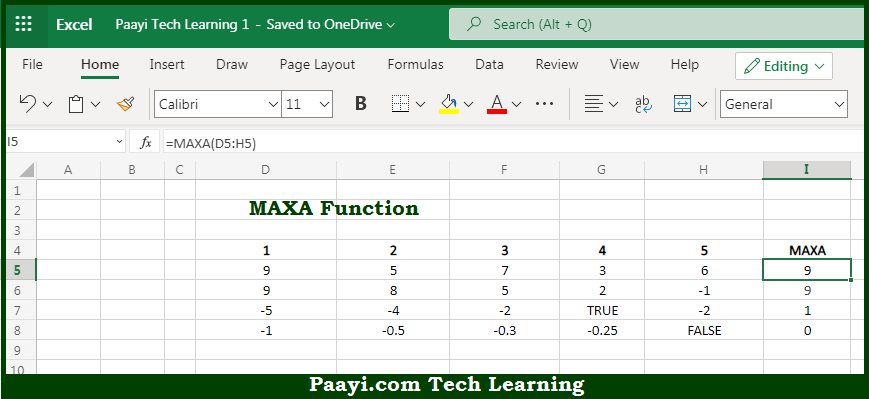# Learn How to Use Microsoft Excel MAXA Function

Written by | 0 Comments | 657 Views

In this article, you will learn how to use the Microsoft Excel MAXA function and its prime function in Microsoft Excel. You will also get to know the Microsoft Excel MAXA function return value and syntax with the help of some examples.

Microsoft Excel MAXA Function

The main purpose of the Microsoft Excel MAXA function is to return the largest value. That implies, with the help of the MAXA function you can able to return the largest numeric values in the range of values. It should be noted that The MAXA function ignores empty cells, the logical values TRUE and FALSE, as 1 and 0 respectively. So, with the help of the MAXA function, you can able to get the largest value in the provided values.

Return Value of MAXA Function

The return value will be the largest numeric value.

Syntax of MAXA Function

=MAXA(value1, [value2], ...)

Where the arguments:

• value1: This is the number, reference to a numeric value or range that contains numeric values.
• value2: This is the number, reference to a numeric value or range that contains numeric values (optional).

## How to Use Microsoft Excel MAXA Function?So we know that Microsoft Excel MAXA function you can able to return the largest value. That implies, with the help of the MAXA function you can able to return the largest numeric values in the range of values. It should be noted that The MAXA function ignores empty cells, the logical values TRUE and FALSE, as 1 and 0 respectively. So, with the help of the MAXA function, you can able to get the largest value in the provided values.